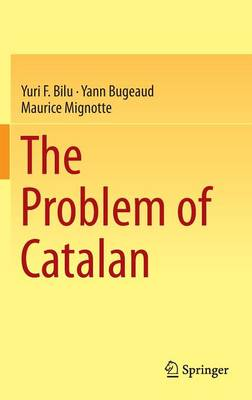•# The Problem of Catalan (Hardback)

(author), (author), (author)
£89.99
Hardback 245 Pages / Published: 27/10/2014
• We can order this

Usually dispatched within 3 weeks

In 1842 the Belgian mathematician Eugene Charles Catalan asked whether 8 and 9 are the only consecutive pure powers of non-zero integers. 160 years after, the question was answered affirmatively by the Swiss mathematician of Romanian origin Preda Mihailescu. In other words, 32 - 23 = 1 is the only solution of the equation xp - yq = 1 in integers x, y, p, q with xy 0 and p, q 2.

In this book we give a complete and (almost) self-contained exposition of Mihailescu's work, which must be understandable by a curious university student, not necessarily specializing in Number Theory. We assume a very modest background:a standard university course of algebra, including basic Galois theory, and working knowledge of basic algebraic number theory.

Publisher: Springer International Publishing AG
ISBN: 9783319100937
Number of pages: 245
Weight: 5148 g
Dimensions: 235 x 155 x 16 mm
Edition: 2014 ed.